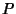## Steiner's Segment Problem

Givenpoints, find the line segments with the shortest possible total length which connect the points. The segments need not necessarily be straight from one point to another.

For three points, if all Angles are less than 120°, then the line segments are those connecting the three points to a central pointwhich makes the Angles,, andall 120°. If one Angle is greater that 120°, thencoincides with the offending Angle.

For four points,is the intersection of the two diagonals, but the required minimum segments are not necessarily these diagonals.

A modified version of the problem is, given two points, to find the segments with the shortest total length connecting the points such that each branch point may be connected to only three segments. There is no general solution to this version of the problem.